# How to Calculate and Solve for Moisture Content | Refractories

The moisture content is illustrated by the image below.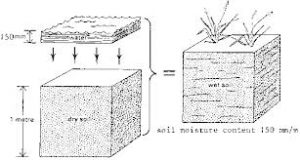To compute for moisture content, two essential parameters are needed and these parameters are Weight of the Sample before Drying (G) and Weight of the Sample after Drying (G1).

The formula for calculating moisture content:

w = 100((G – G1)/G)

Where:

w = Moisture Content
G = Weight of the Sample before Drying
G1 = Weight of the Sample after Drying

Let’s solve an example;
Find the moisture content when the weight of the sample before drying is 40 and the weight of the sample after drying is 10.

This implies that;

G = Weight of the Sample before Drying = 40
G1 = Weight of the Sample after Drying = 10

w = 100((G – G1)/G)
w = 100((40 – 10)/40)
w = 100(30/40)
w = 100(0.75)
w = 75

Therefore, the moisture content is 75%.

Nickzom Calculator – The Calculator Encyclopedia is capable of calculating the moisture content.

To get the answer and workings of the moisture content using the Nickzom Calculator – The Calculator Encyclopedia. First, you need to obtain the app.

You can get this app via any of these means:

You can also try the demo version via https://www.nickzom.org/calculator

Apple (Paid) – https://itunes.apple.com/us/app/nickzom-calculator/id1331162702?mt=8
Once, you have obtained the calculator encyclopedia app, proceed to the Calculator Map, then click on Materials and Metallurgical under Engineering.Now, Click on Refractories under Materials and Metallurgical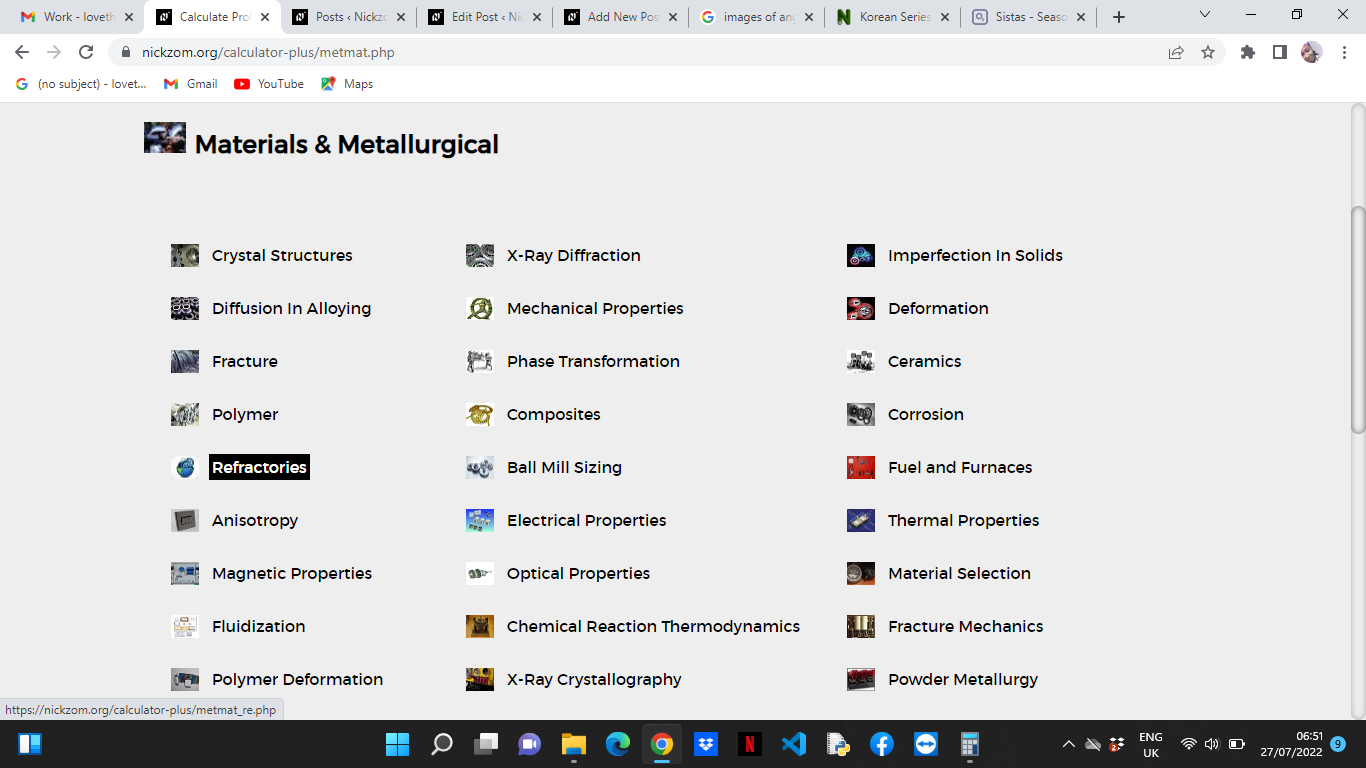Now, Click on Moisture Content under Refractories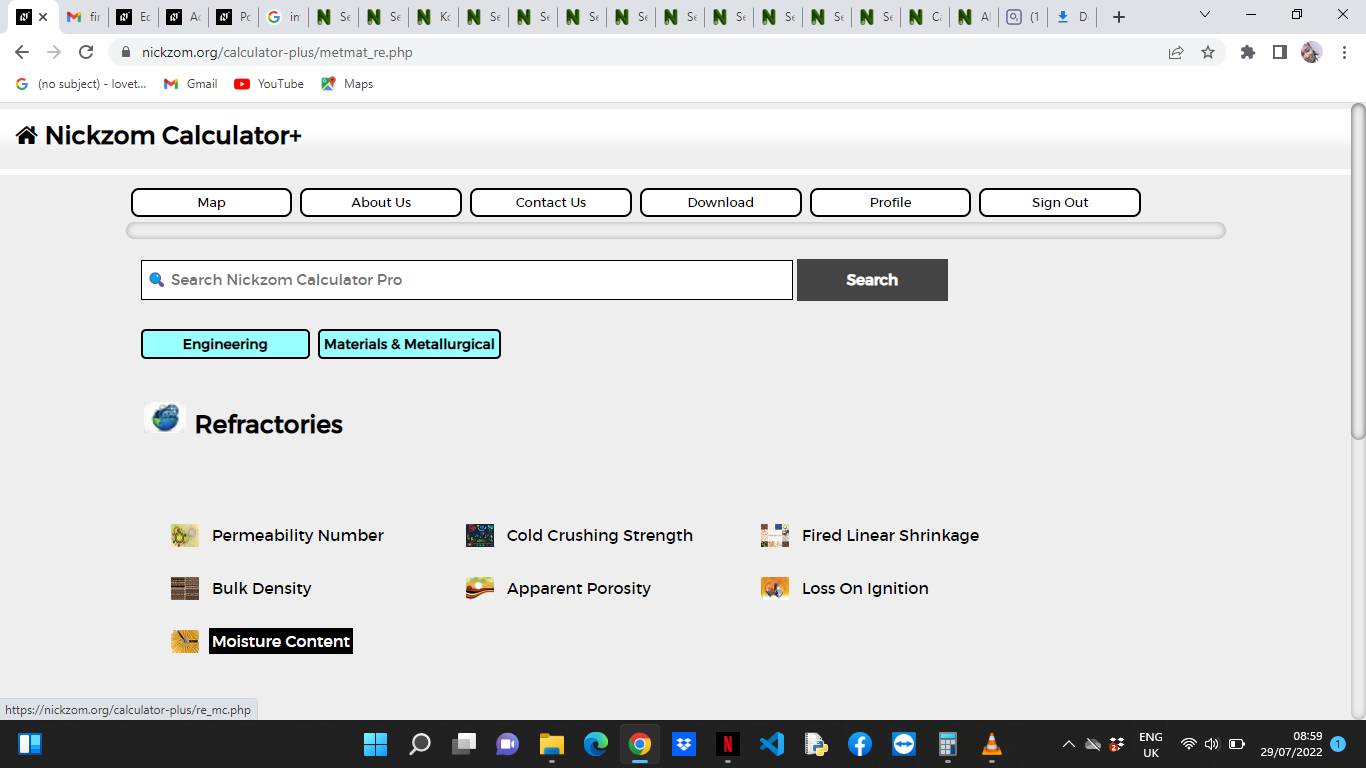The screenshot below displays the page or activity to enter your values, to get the answer for the moisture content according to the respective parameter which is the Weight of the Sample before Drying (G) and Weight of the Sample after Drying (G1).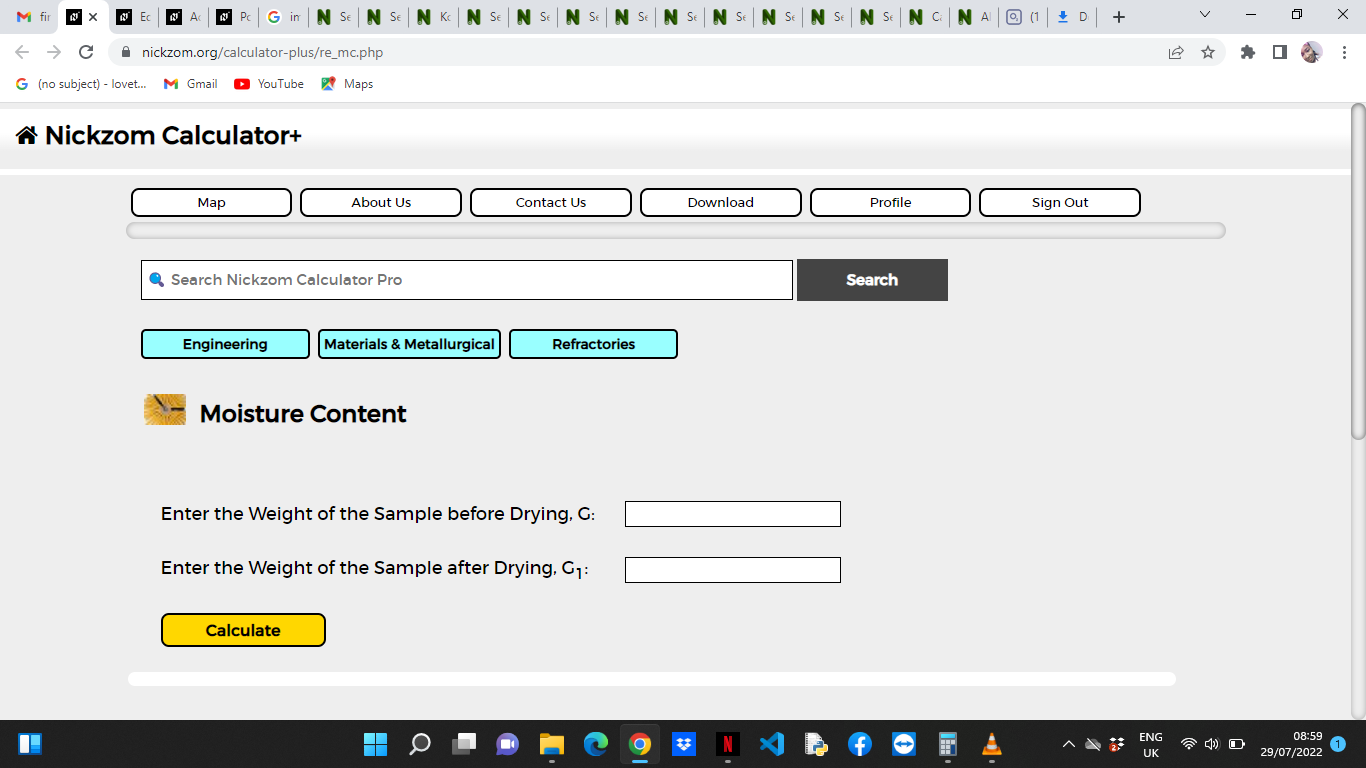Now, enter the values appropriately and accordingly for the parameters as required by the Weight of the Sample before Drying (G) is 40 and Weight of the Sample after Drying (G1) is 10.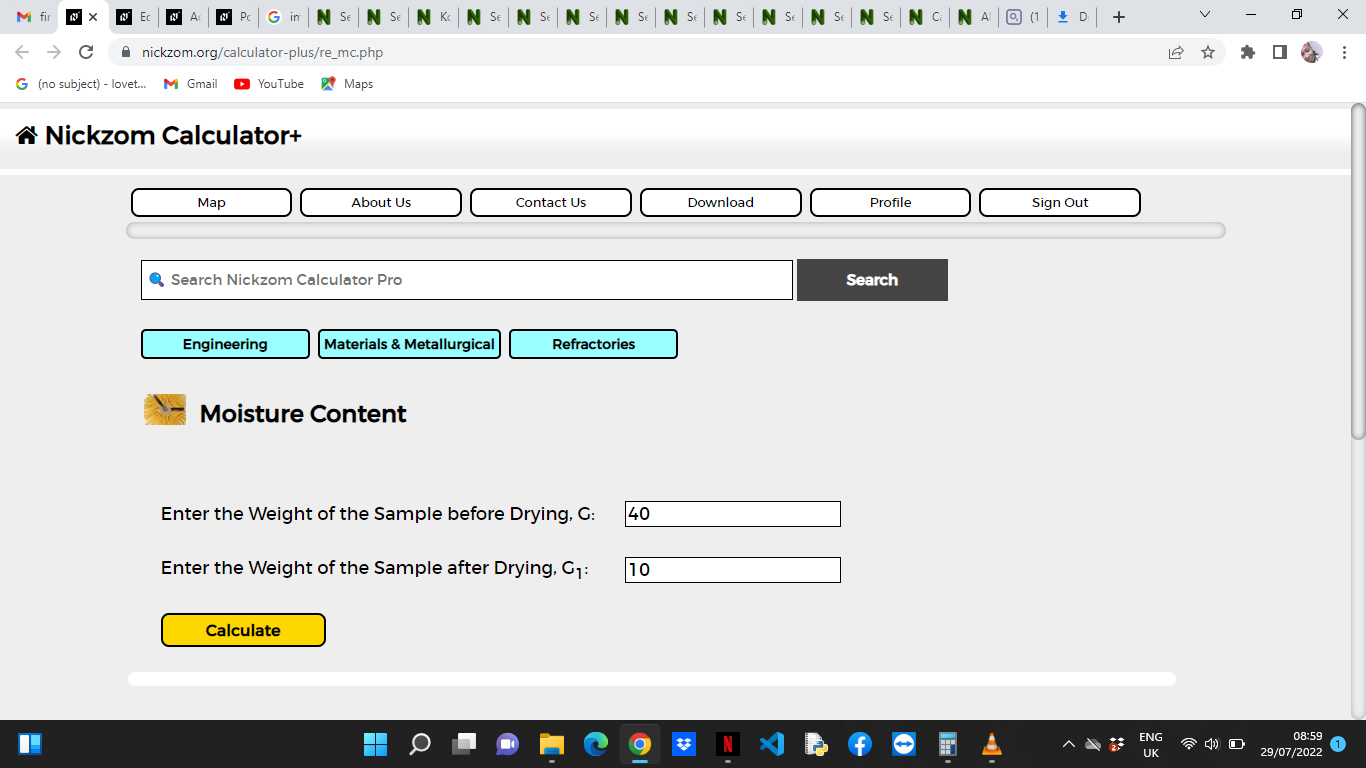Finally, Click on Calculate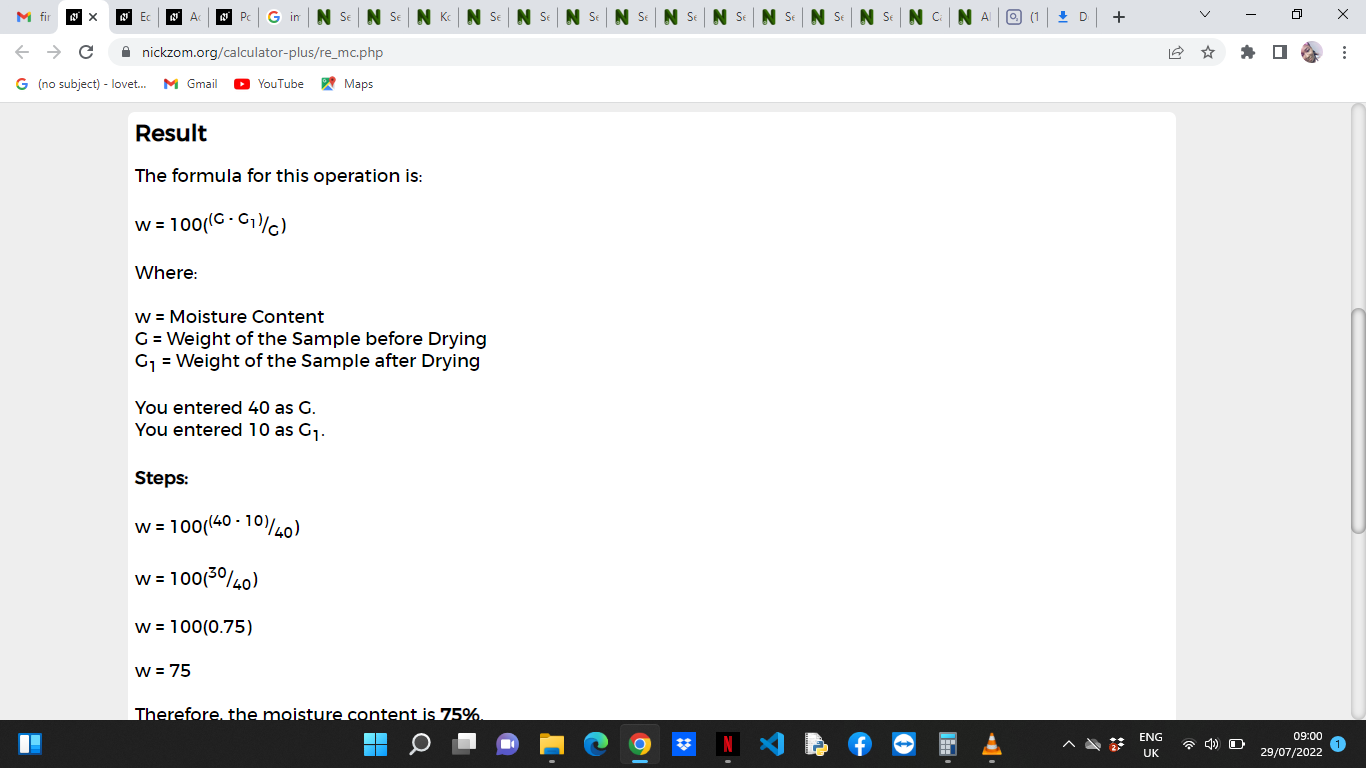As you can see from the screenshot above, Nickzom Calculator– The Calculator Encyclopedia solves for the moisture content and presents the formula, workings and steps too.